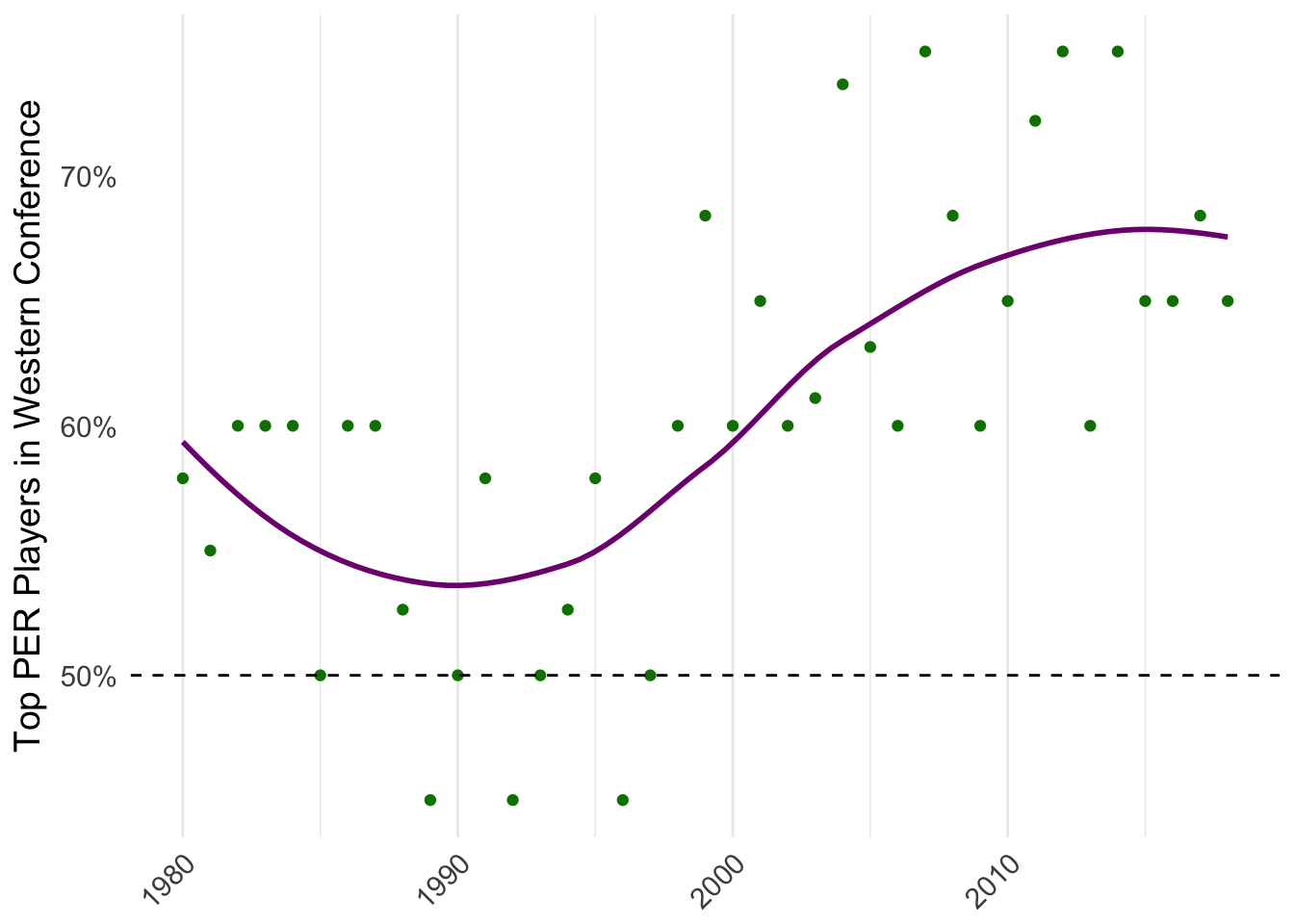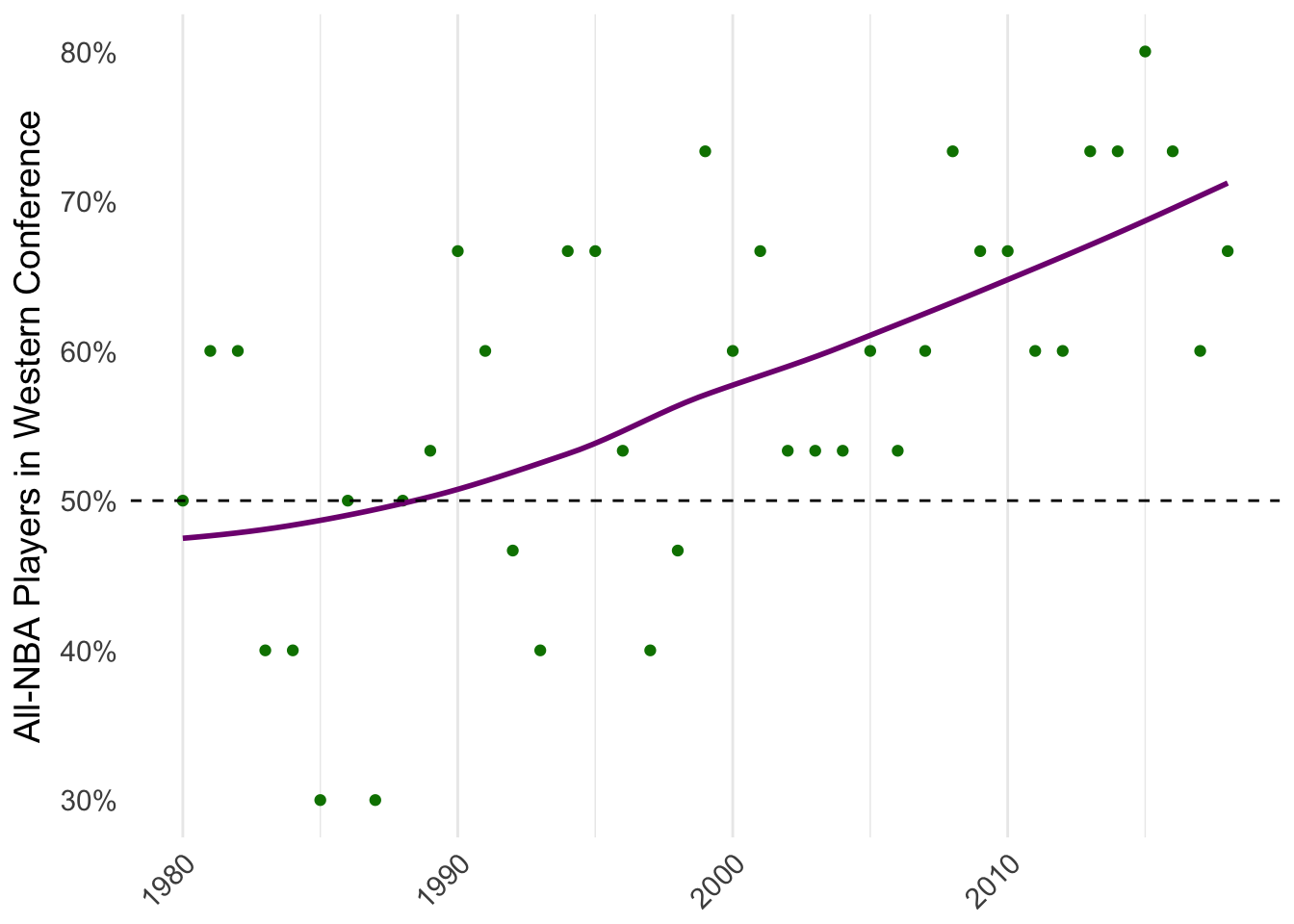# In Support of Open Seeding in the NBA, Pt. 2

This is my second post providing support for open seeding in the NBA; this would mean that the top 16 teams in the league make the playoffs (instead of the top 8 in each conference). Last time, I showed that in only 10 of the last 34 seasons have the 16 teams with the best records been the 16 playoff teams. I wanted to look more at the player level, particularly after big names have recently migrated from the the Eastern to the Western Conference. It feels like there is a huge conference imbalance in star power in the league at a time when individual stars mean a lot to the game.

I again scraped Basketball-Reference.com for the numbers, and the code can be found at the end of this post. Basketball-Reference has league leaders pages, listing the top 20 players for a season in various statistics. I consider player efficiency rating (PER) to be the best overall performance metric, so I calculated what percent of the top 20 PER performers were in the West for every season since the 1979-1980 season. (I chose to start in this season because the league leaders pages look slightly different in previous seasons, making it more difficult to scrape). If the conferences were balanced, we would expect this to be 50%. I also did the same thing for All-NBA teams: What proportion of the All-NBA players were from the Western Conference? Again, we would expect this number to be 50% if the conferences were balanced perfectly.

``````results_per %>%
filter(complete.cases(.)) %>%
group_by(year) %>%
count(conf) %>%
mutate(prop = n / sum(n)) %>%
ungroup() %>%
filter(conf == "W") %>%
ggplot(aes(x = as.numeric(year), y = prop)) +
geom_point(color = "#008000") +
stat_smooth(se = FALSE, method = "loess", span = 1, color = "#800080") +
theme_minimal() +
theme(
axis.text.x = element_text(hjust = 1, angle = 45),
panel.grid.major.y = element_blank(),
panel.grid.minor.y = element_blank(),
text = element_text(size = 14)
) +
labs(x = NULL) +
scale_y_continuous(
name = "Top PER Players in Western Conference",
label = function(x) paste0(x * 100, "%")
) +
geom_hline(yintercept = .5, linetype = 2)````````````results_allnba %>%
filter(complete.cases(.)) %>%
group_by(year) %>%
filter(conf == "W") %>%
ggplot(aes(x = year, y = prop, group = 1)) +
geom_point(color = "#008000") +
stat_smooth(se = FALSE, method = "loess", span = 1, color = "#800080") +
theme_minimal() +
theme(
axis.text.x = element_text(hjust = 1, angle = 45),
panel.grid.major.y = element_blank(),
panel.grid.minor.y = element_blank(),
text = element_text(size = 14)
) +
labs(x = NULL) +
scale_y_continuous(
name = "All-NBA Players in Western Conference",
label = function(x) paste0(x * 100, "%")
) +
geom_hline(yintercept = .5, linetype = 2)``````Western conference players have been overrepresented in the Western Conference in most years. This means the NBA—perhaps the most star-driven league in North American sports—doesn’t have the opportunity to showcase all of their great players in the playoffs, because only 8 teams from the West are allowed to compete there. It also looks as though this imbalance is at an all-time high, which could be why talk about open seeding has reached mainstream support and discussion among thinkers around the league recently. Allowing the top 16 teams to play in the playoffs would allow more stars to play on that national stage.

## R Code Appendix

Scraping this posed a fun problem, because a few of the tables I was trying to access were written as comments in the HTML code for the webpage. This meant that I could not just run `rvest::html_table` and choose the ones I was looking for. Instead, it meant that I had to find the XPath to that comment, scrape it, convert it to a character string, read the string in again as HTML, and then parse the table out. This code also shows how one can efficiently vectorize using an `apply` function and then piping into a `do.call` command to bind the results together into a `data.frame`.

The entirety of the code for this post can be found at my GitHub page.

``````library(tidyverse)
library(rvest)
years <- 1980:2018
per <- lapply(years, function(x) {
paste0(
x,
) %>%
html_table() %>%
getElement(30) %>%
transmute(
team = substr(X2, nchar(X2) - 2, nchar(X2)),
per = X3
)
})
names(per) <- years

standings <- lapply(years, function(x) {
tmp <- paste0(
x,
"_ratings.html"
) %>%
html_table() %>%
getElement(1) %>%
`[`(-1, 2:3)
colnames(tmp) <- c("team", "conf")
tmp
})
names(standings) <- years

key <- lapply(years, function(x) {
tmp <- paste0(
x,
"_standings.html"
) %>%
html_node(xpath = '//*[@id="all_team_vs_team"]/comment()') %>%
html_text() %>%
html_table() %>%
getElement(1) %>%
as.data.frame()

suppressWarnings(
tmp <- data.frame(
team = tmp\$Team,
abbr = colnames(tmp)[-1:-2]
) %>%
full_join(standings[[as.character(x)]], by = "team") %>%
select("abbr", "conf")
)

colnames(tmp)[] <- "team"
tmp
})
names(key) <- years

results_per <- lapply(as.character(years), function(x) {
tmp <- suppressWarnings(left_join(per[[x]], key[[x]], by = "team"))
tmp\$year <- x
tmp
}) %>%
do.call(rbind, .)

results_allnba <- lapply(years, function(x) {
tmp <- paste0(
x,
"_per_game.html"
) %>%
html_table() %>%
getElement(1) %>%
mutate(Player = gsub("*", "", Player, fixed = TRUE)) %>%
group_by(Player) %>%
slice(1) %>%
ungroup() %>%
transmute(player = Player, team = Tm)

suppressWarnings(
paste0(
x,
".html#all_all-nba"
) %>%
html_node(xpath = '//*[@id="all_all-nba"]/comment()') %>%
html_text() %>%